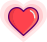### RSA 算法基础知识

#### 密码学知识

（1）甲方选择某一种加密规则，对信息进行加密；
（2）乙方使用同一种规则，对信息进行解密。

（1）乙方生成两把密钥（公钥和私钥）。公钥是公开的，任何人都可以获得，私钥则是保密的。
（2）甲方获取乙方的公钥，然后用它对信息加密。
（3）乙方得到加密后的信息，用私钥解密。

### RSA 算法流程

（1）选择两个不相等的质数p和q

（2）计算p和q的乘积n

n = p*q = 61 * 53 = 3233。

（3）计算 n 的欧拉函数 φ(n)

φ(3233) = φ(61 * 53) = φ(61) * φ(53)。

（4）随机选择一个整数 e ，条件是1< e < φ(n)，且 e 与 φ(n) 互质

（5）计算e对于φ(n)的模反元素d
e * d ≡ 1 (mod φ(n))。其中e = 17，φ(n) = 3120。

17 * d = 3120k + 1。继续转化得到：
17 * d + 3120y = 1。其中y = -k。

（6）将n和e封装成公钥，n和d封装成私钥

### RSA 算法分析

（1）ed ≡ 1 (mod φ(n))。只有知道 e 和 φ(n)，才能算出 d 。
（2）φ(n) = (p-1)(q-1)。只有知道 p 和 q ，才能算出 φ(n)。

### RSA 算法总结

RSA 的安全性依赖于大数分解，因此 RSA 算法加密安全性较高。但是，RSA 算法为保证安全性，会大大提升密钥长度，导致运算速度变慢。这导致它在大量数据加密时并不适用。

##原 创 热 文 推 荐